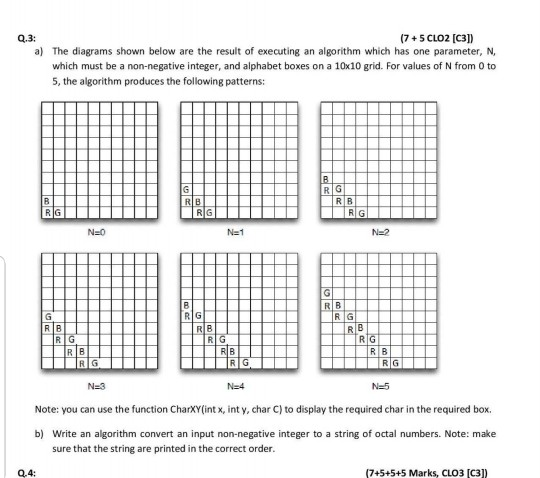## a) The diagrams shown below are the result of executing an algorithm which has one paramet...a) The diagrams shown below are the result of executing an algorithm which has one parameter, N, which must be a non-negative integer, and alphabet boxes on a 10<10 grid. For values of N from O to 5, the algorithm produces the following patterns:

Solved
COMPUTER SCIENCE 1 Answer Hanzel Paz